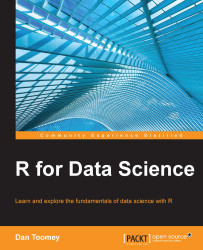•#### R for Data Science#### Overview of this book

R for Data ScienceCreditswww.PacktPub.comPrefaceFree Chapter
Data Mining PatternsData Mining SequencesText MiningData Analysis – Regression AnalysisData Analysis – CorrelationData Analysis – ClusteringData Visualization – R GraphicsData Visualization – PlottingData Visualization – 3DMachine Learning in ActionPredicting Events with Machine LearningSupervised and Unsupervised LearningIndex## Scatter plots

A scatter plot is a basic plotting device comparing datasets with two axes. A basic scatter plot is provided with the `plot` function built into the R system.

### Note

Several objects available in R and packages have their own `plot` function in order to effectively portray the data associated.

The `plot` function looks as follows:

```plot(x,
y,
type,
main,
sub,
xlab,
ylab,
asp)```

The various parameters of this function are described in the following table:

Parameter

Description

`x`

This is an independent variable.

`y`

This is a dependent variable.

`type`

This defines the type of plot. It should be one of the following types:

• `p` for points

• `l` for lines

• `b` for both

• `c` for the lines part alone of `b`

• `o` for both overplotted

• `h` for histogram-like (or high-density) vertical lines

• `s` for stair steps

• `S` for other steps, see details below

• `n` for no plotting (not sure why this is a choice as it ends up with no information plotted)

`main`

This is the title of the plot.

`sub`

This...